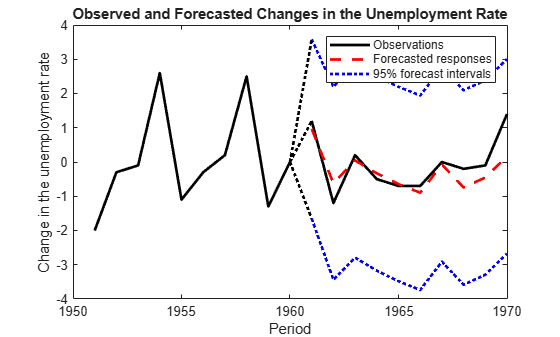# Forecast Observations of State-Space Model Containing Regression Component

This example shows how to estimate a regression model containing a regression component, and then forecast observations from the fitted model.

Suppose that the linear relationship between the change in the unemployment rate and the nominal gross national product (nGNP) growth rate is of interest. Suppose further that the first difference of the unemployment rate is an ARMA(1,1) series. Symbolically, and in state-space form, the model is

`$\begin{array}{l}\left[\begin{array}{c}{x}_{1,t}\\ {x}_{2,t}\end{array}\right]=\left[\begin{array}{cc}\varphi & \theta \\ 0& 0\end{array}\right]\left[\begin{array}{c}{x}_{1,t-1}\\ {x}_{2,t-1}\end{array}\right]+\left[\begin{array}{c}1\\ 1\end{array}\right]{u}_{1,t}\\ {y}_{t}-\beta {Z}_{t}={x}_{1,t}+\sigma {\epsilon }_{t},\end{array}$`

where:

• ${x}_{1,t}$ is the change in the unemployment rate at time t.

• ${x}_{2,t}$ is a dummy state for the MA(1) effect.

• ${y}_{1,t}$ is the observed change in the unemployment rate being deflated by the growth rate of nGNP (${Z}_{t}$).

• ${u}_{1,t}$ is the Gaussian series of state disturbances having mean 0 and standard deviation 1.

• ${\epsilon }_{t}$ is the Gaussian series of observation innovations having mean 0 and standard deviation $\sigma$.

Load the Nelson-Plosser data set, which contains the unemployment rate and nGNP series, among other things.

`load Data_NelsonPlosser`

Preprocess the data by taking the natural logarithm of the nGNP series, and the first difference of each series. Also, remove the starting `NaN` values from each series.

```isNaN = any(ismissing(DataTable),2); % Flag periods containing NaNs gnpn = DataTable.GNPN(~isNaN); u = DataTable.UR(~isNaN); T = size(gnpn,1); % Sample size Z = [ones(T-1,1) diff(log(gnpn))]; y = diff(u);```

Though this example removes missing values, the software can accommodate series containing missing values in the Kalman filter framework.

To determine how well the model forecasts observations, remove the last 10 observations for comparison.

```numPeriods = 10; % Forecast horizon isY = y(1:end-numPeriods); % In-sample observations oosY = y(end-numPeriods+1:end); % Out-of-sample observations ISZ = Z(1:end-numPeriods,:); % In-sample predictors OOSZ = Z(end-numPeriods+1:end,:); % Out-of-sample predictors```

Specify the coefficient matrices.

```A = [NaN NaN; 0 0]; B = [1; 1]; C = [1 0]; D = NaN;```

Specify the state-space model using `ssm`.

`Mdl = ssm(A,B,C,D);`

Estimate the model parameters. Specify the regression component and its initial value for optimization using the `'Predictors'` and `'Beta0'` name-value pair arguments, respectively. Restrict the estimate of $\sigma$ to all positive, real numbers. For numerical stability, specify the Hessian when the software computes the parameter covariance matrix, using the `'CovMethod'` name-value pair argument.

```params0 = [0.3 0.2 0.1]; % Chosen arbitrarily [EstMdl,estParams] = estimate(Mdl,isY,params0,'Predictors',ISZ,... 'Beta0',[0.1 0.2],'lb',[-Inf,-Inf,0,-Inf,-Inf],'CovMethod','hessian');```
```Method: Maximum likelihood (fmincon) Sample size: 51 Logarithmic likelihood: -87.2409 Akaike info criterion: 184.482 Bayesian info criterion: 194.141 | Coeff Std Err t Stat Prob ---------------------------------------------------------- c(1) | -0.31780 0.19429 -1.63572 0.10190 c(2) | 1.21242 0.48882 2.48032 0.01313 c(3) | 0.45583 0.63930 0.71302 0.47584 y <- z(1) | 1.32407 0.26313 5.03201 0 y <- z(2) | -24.48733 1.90115 -12.88024 0 | | Final State Std Dev t Stat Prob x(1) | -0.38117 0.42842 -0.88971 0.37363 x(2) | 0.23402 0.66222 0.35339 0.72380 ```

`EstMdl` is an `ssm` model, and you can access its properties using dot notation.

Forecast observations over the forecast horizon. `EstMdl` does not store the data set, so you must pass it in appropriate name-value pair arguments.

```[fY,yMSE] = forecast(EstMdl,numPeriods,isY,'Predictors0',ISZ,... 'PredictorsF',OOSZ,'Beta',estParams(end-1:end));```

`fY` is a 10-by-1 vector containing the forecasted observations, and `yMSE` is a 10-by-1 vector containing the variances of the forecasted observations.

Obtain 95% Wald-type forecast intervals. Plot the forecasted observations with their true values and the forecast intervals.

```ForecastIntervals(:,1) = fY - 1.96*sqrt(yMSE); ForecastIntervals(:,2) = fY + 1.96*sqrt(yMSE); figure h = plot(dates(end-numPeriods-9:end-numPeriods),isY(end-9:end),'-k',... dates(end-numPeriods+1:end),oosY,'-k',... dates(end-numPeriods+1:end),fY,'--r',... dates(end-numPeriods+1:end),ForecastIntervals,':b',... dates(end-numPeriods:end-numPeriods+1),... [isY(end)*ones(3,1),[oosY(1);ForecastIntervals(1,:)']],':k',... 'LineWidth',2); xlabel('Period') ylabel('Change in the unemployment rate') legend(h([1,3,4]),{'Observations','Forecasted responses',... '95% forecast intervals'}) title('Observed and Forecasted Changes in the Unemployment Rate')```This model seems to forecast the changes in the unemployment rate well.